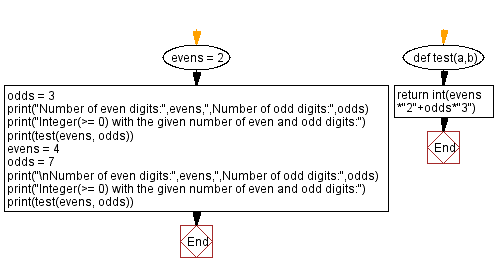﻿ Python: Find an integer with the given number of even and odd digits - w3resource# Python: Find an integer with the given number of even and odd digits

## Python Programming Puzzles: Exercise-88 with Solution

Write a Python program to find an integer (n >= 0) with the given number of even and odd digits.

```Input:
Number of even digits: 2 ,Number of odd digits: 3
Output:
22333

Input:
Number of even digits: 4 ,Number of odd digits: 7
Output:
22223333333
```

Sample Solution:

Python Code:

``````#License: https://bit.ly/3oLErEI

def test(a,b):
return int(evens*"2"+odds*"3")
evens = 2
odds = 3
print("Number of even digits:",evens,",Number of odd digits:",odds)
print("Integer(>= 0) with the given number of even and odd digits:")
print(test(evens, odds))
evens = 4
odds = 7
print("\nNumber of even digits:",evens,",Number of odd digits:",odds)
print("Integer(>= 0) with the given number of even and odd digits:")
print(test(evens, odds))
``````

Sample Output:

```Number of even digits: 2 ,Number of odd digits: 3
Integer(>= 0) with the given number of even and odd digits:
22333

Number of even digits: 4 ,Number of odd digits: 7
Integer(>= 0) with the given number of even and odd digits:
22223333333
```

Flowchart:## Visualize Python code execution:

The following tool visualize what the computer is doing step-by-step as it executes the said program:

Python Code Editor :

Have another way to solve this solution? Contribute your code (and comments) through Disqus.

What is the difficulty level of this exercise?

Test your Programming skills with w3resource's quiz.

﻿

## Python: Tips of the Day

Clamps num within the inclusive range specified by the boundary values x and y:

Example:

```def tips_clamp_num(num,x,y):
return max(min(num, max(x, y)), min(x, y))
print(tips_clamp_num(2, 4, 6))
print(tips_clamp_num(1, -1, -6))
```

Output:

```4
-1
```